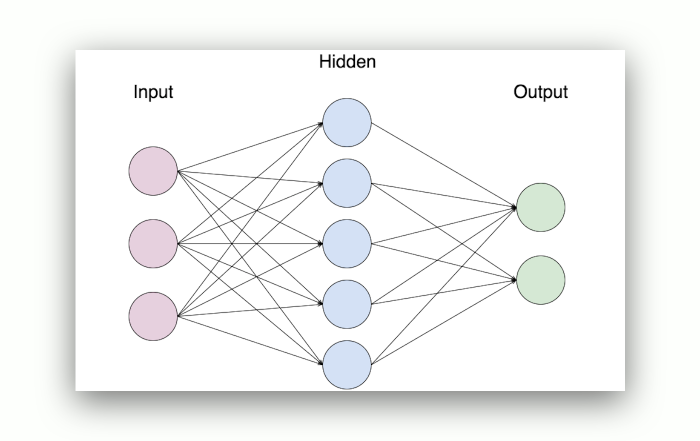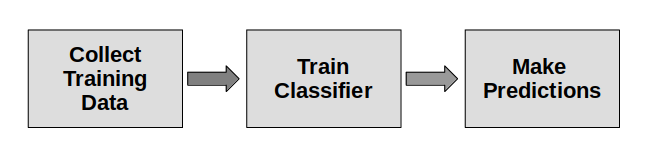tag: deep learning | Python Tutorial

# Tag: deep learning

## Multilayer Perceptron

Related Course:
Deep Learning for Computer Vision with Tensor Flow and KerasA perceptron represents a simple algorithm meant to perform binary classification or simply put: it established whether the input belongs to a certain category of interest or not.

Moreover, it is rather important in the history of neural networks and artificial intelligence due to the fact that it was characterized by Frank Rosenblatt as a device rather than an algorithm.

A perceptron represents a linear classifier that is able to classify input by separating two categories with a line.

Thus, the input is usually viewed as a feature vector X multiplied by weights W and added to a bias B: y=W * x + b.

This classifier delivers a unique output based on various real-valued inputs by setting up a linear combination based on its input weights.

## Single vs Multi-Layer perceptrons

Rosenblatt set up a single-layer perceptron a hardware-algorithm that did not feature multiple layers, but which allowed neural networks to establish a feature hierarchy. Hence, it represented a vague neural network, which did not allow his perceptron to perform non-linear classification.

On the other hand, a multilayer perceptron or MLP represents a vast artificial neural network, meaning simply that it features more than one perceptron. This gathering of perceptrons is established from an input layer meant to receive the signal, an output layer responsible for a decision or prediction in regards to the input, and an arbitrary number of hidden layers that represent the true computational power of the MLP.

Usually, multilayer perceptrons are used in supervised learning issues due to the fact that they are able to train on a set of input-output pairs and learn to depict the dependencies between those inputs and outputs.

Training requires the adjustment of parameters of the model with the sole purpose of minimizing error.

Furthermore, backpropagation is required to make those weigh and bias adjustments, while the error resulted can be established in a multitude of ways, such as the root mean squared error or RMSE.

MLPs are basically linked to two motions, one that goes back and one that goes forth. In the forward phase, the signal travels from the input layer through the hidden layers towards the output layer, while the decision of the output layer is established in regards to the ground truth labels. In the backwards pass, the multitude of weights and biases are backpropagated through the MLP. The landscape of error is provided by differentiation, which can be done by making use of any gradient-based optimisation algorithm.

## Multi-layer perceptron sklearn

A multi-layer perceptron model is able to solve any problem, but it is still difficult to understand if you compare it to other models more user-friendly such as linear regression.

## Perceptron

You wake up, look outside and see that it is a rainy day. The clock marks 11:50 in the morning, your stomach starts rumbling asking for food and you don’t know what you are having for lunch.

You go to the kitchen, open the fridge and all you can find is an egg, a carrot and an empty pot of mayonnaise. You don’t want to go out in the rain to a restaurant, so what do you do? Seems like the best answer is ordering food!

Every time we think about what decision to make, we are weighing down the options at hand. Instinctively, without realizing, our brain assigns different values to some of the variables so we are able to decide properly.

In the example above, ordering food was the best alternative because it would be faster (hunger aspect), make up for the lack of ingredients in the house and not make you go out in the rain.

Frank Rosenblatt was able to put this into mathematical terms back in the late 50’s creating the first and most simple type of Artificial Neural Network, known as the Perceptron.

Related Course:
Deep Learning for Computer Vision with Tensor Flow and Keras## Perceptron

It works as an artificial neuron with a basic form of activation: a simple binary formula called Heaviside Step function that has only two possible results: 1 and 0.

The way the Perceptron calculates the result is by adding all the inputs multiplied by their own weight value, which express the importance of the respective inputs to the output.

An offset (called bias) is then added to the weighted sum and if the input is negative or zero, the output is 0. However, for any positive input, the output will be 1.

The training process of a Perceptron consists on making the model learn the ideal values of weights and biases, presenting to the model the input data and the possible outputs.

During the training, weights and biases are learned. Now, with the trained model, we can present new input data and the model will be able to predict the output.

## sklearn perceptron

Even though the Perceptron is the simplest type of artificial neural network, it can be used in supervised learning and to classify the input data provided.

## Introduction to Neural Networks

Neural networks are inspired by the brain. The model has many neurons (often called nodes). We don’t need to go into the details of biology to understand neural networks.

Like a brain, neural networks can “learn”. Instead of learning, the term “training” is used. If training is completed, the system can make predictions (classifications).

Related Course:
Deep Learning for Computer Vision with Tensor Flow and Keras## Introduction

The neural network has: an input layer, hidden layers and an output layer. Each layer has a number of nodes.

The nodes are connected and there is a set of weights and biases between each layer (W and b).

There’s also an activation function for each hidden layer, σ. You can use the sigmoid activation function.When couting the layers of a network, the input layer is often not counted. If we say 2-layer neural network, it means th
ere are 3 layers.

To explain better, we’ll add some sample code in this tutorial.

In code:

## Layers

The layers are connected. The first layer is made with the input layer and weights. In this case the output layer is made with layer1 and weights2.

For a 2 layer neural network, you can have this:

## Training

Remember we said neural networks have a training process?

The training process has multiple iterations. Each iteration

• calculate the predicted output y (feedforward)
• updates the weights and biases (backpropagation)

During the feedforward propagation process (see code above), it uses the weights to predict the output.
But what is a good output?

To find out, you need a loss function (frequently called cost function). There are many loss functions.

The loss function will be used to update the weights and biases. It’s part of the backpropagation process.

## Deep LearningDeep Learning is all exciting! Deep Learning can be used for making predictions, which you may be familiar with from other Machine Learning algorithms.

Becoming good at Deep Learning opens up new opportunities and gives you a big competitive advantage. You can do way more than just classifying data..

Related Course: Deep Learning A-Z™: Hands-On Artificial Neural Networks## Deep Learning Applications

Deep Learning can be applied in many industries: Consumer, Industry, Art, Finance, Science, Robotics, Energy, Transportation and more.

Applications of Deep Learning in the real world are:
 Task Details Recognizing faces Recognize faces in images or videos Object Recognition Neural Networks can recognize objects in images, in fact, better than humans. Caption Generation Given input of an image, it can output a text describing whats happening in the image Deep Speech All of the big companies have a Speech Recognition system that is based on Deep Learning. Given a sound file or real time sound, the software can convert it to text. Language Translation Given an input language, say English, a neural network can translate it to another language in a natural way. Not just that: think real-time translation. Data Centers Optimize cooling of data centers. Google used it to save billions of dollars based on an algorithm! Medical: Automated Detection Automated Detection of Retinal Disease or other diseases Self driving cars Complete autonomous driving

## Neural Network Example

### Neural Network Example

In this article we’ll make a classifier using an artificial neural network.
While internally the neural network algorithm works different from other supervised learning algorithms, the steps are the same:Related course:

## Training data

 Array Contains Size X training samples represented as floating point feature vectors size (n_samples, n_features) y class labels for the training samples size (n_samples,)

In code we define that as:

## Train classifier

We then create the classifier:

Train the classifier with training data:

## Predict

And finally we can make predictions:

The neural network code is then: